# Test: Moving Charges And Magnetism 1 - From Past 28 Years Questions

## 20 Questions MCQ Test Physics 28 Years Past year papers for NEET/AIPMT Class 12 | Test: Moving Charges And Magnetism 1 - From Past 28 Years Questions

Description
This mock test of Test: Moving Charges And Magnetism 1 - From Past 28 Years Questions for Class 12 helps you for every Class 12 entrance exam. This contains 20 Multiple Choice Questions for Class 12 Test: Moving Charges And Magnetism 1 - From Past 28 Years Questions (mcq) to study with solutions a complete question bank. The solved questions answers in this Test: Moving Charges And Magnetism 1 - From Past 28 Years Questions quiz give you a good mix of easy questions and tough questions. Class 12 students definitely take this Test: Moving Charges And Magnetism 1 - From Past 28 Years Questions exercise for a better result in the exam. You can find other Test: Moving Charges And Magnetism 1 - From Past 28 Years Questions extra questions, long questions & short questions for Class 12 on EduRev as well by searching above.
QUESTION: 1

### The magnetic force acting on a charged particleof charge – 2μC in a magnetic field of 2T actingin y direction, when the particle velocity is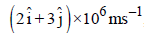, is

Solution:

The magnetic force acting on the char ged paraticle is given by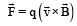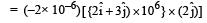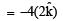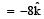∴  Force is of 8N along – z-axis.

QUESTION: 2

### Under the influence of a uniform magnetic field,a charged particle moves with constant speed vin a circle of radius R. The time period of rotationof the particle: 

Solution:

The time period of the charged particle is
given by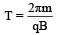Thus, time period is independent of both v and R.

QUESTION: 3

### A thin ring of radius R meter has charge q coulomb uniformly spread on it. The ring rotates about its axis with a constant frequency of f revolutions/s. The value of magnetic induction in Wb/m2 at the centre of the ring is 

Solution:

Current in the ring due to rotation,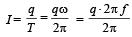Therefore, magnetic field at the centre of the ring is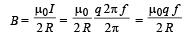QUESTION: 4

A galvanometer has a coil of resistance 100 ohmand gives a full-scale deflection for 30 mAcurrent. It is to work as a voltmeter of 30 voltrange, the resistance required to be added willbe           

Solution:

Let the resistance to be added be R, then

30 = Ig(r + R)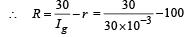= 1000 – 100 = 900Ω

QUESTION: 5

A square current carrying loop is suspended in a uniform magnetic field acting in the plane of the loop. If the force on one arm of the loop is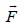, the net force on the remaining three arms of the loop is

Solution:

The force on the two arms parallel to the field is zero.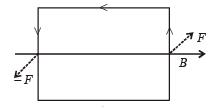∴ Force on remaining arms = – F

QUESTION: 6

A particle having a mass of 10–2 kg carries a charge of 5 × 10–C. The particle is given an initial horozontal velocity of 105 ms–1 in the presence of electric field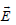and magnetic field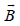. To  keep the particle moving in a horizontal direction, it is necessary that

(1)should be perpendicular to the direction of velocity andshould be along the direction of velocity.

(2) Bothandshould be along the direction of velocity.

(3) Bothandare mutually perpendicular and perpendicular to the direction of velocity.

(4)should be along the direction of velocity andshould be perpendicular to the direction of velocity

Which one of the following pairs of statements is possible?

Solution:

Force due to electric field acts along the direction of the electric field but force due to the magnetic field acts along a direction perpendicular to both the velocity of the charged particle and the magnetic field.
Hence both statements (2) and (3) are true.
In statement (2), magnetic force is zero, so, electric force will keep the particle continue to move in horizontal direction. In statement (3), both electric and magnetic forces will be opposite to each other. If their magnitudes will be equal then the particle will continue horizontal motion.

QUESTION: 7

A closely wound solenoid of 2000 turns and areaof cross-section 1.5 × 10–4 m2 carries a currentof 2.0 A. It suspended through its centre andperpendicular to its length, allowing it to turn ina horizontal plane in a uniform magnetic field 5 ×10–2 tesla making an angle of 30° with the axis ofthe solenoid. The torque on the solenoid will be:

Solution:

Torque on the solenoid is given by ζ = MB sinθ where θ is the angle between the magnetic field and the axis of solenoid.
M = niA
∴   ζ = niA B sin 30°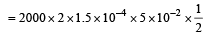= 1.5 x 10-2 N-m

QUESTION: 8

A current loop consists of two identical semicircular parts each of radius R, one lying in the x-y plane and the other in x-z plane. If the current in the loop is i., the resultant magnetic field due to the two semicircular parts at their common centre is

Solution:

Magnetic fields due to the two parts at their common centre are respectively,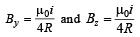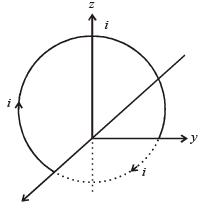Resultant field =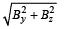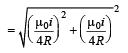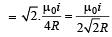QUESTION: 9

A current carrying loop in the form of a right angle isosceles triangle ABC is placed in a uniform magnetic field acting along AB. If the magnetic force on the arm BC is F, what is the force on the arm AC?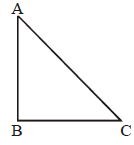Solution:

Let a current i be flowing in the loop ABC in the direction shown in the figure. If the length of each of the sides AB and BC be x then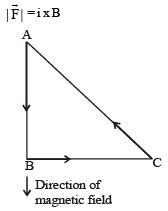where B is the magnitude of the magnetic force.
The direction of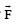will be in the direction perpendicular to the plane of the paper and going into it.
By Pythagorus theorem,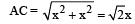∴ Magnitude of force on AC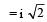x  B sin 45°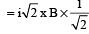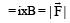The direction of the force on AC is perpendicular to the plane of the paper and going out of it. Hence, force on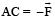QUESTION: 10

A uniform electric field and uniform magneticfield are acting along the same direction in acertain region. If an electron is projected in theregion such that its velocity is pointed alongthe direction of fields, then the electron        

Solution: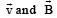are in same direction so that magnetic force on electron becomes zero, only electric force acts. But force on electron due to electric field is opposite to the direction of velocity.

QUESTION: 11

A galvanometer of resistance, G is shunted by a resistance S ohm. To keep the main current in the circuit unchanged, the resistance to be put in series with the galvanometer is          [2011M]

Solution:

To keep the main current in the circuit unchanged, the resistance of the galvanometer should be equal to the net resistance.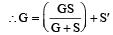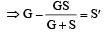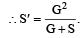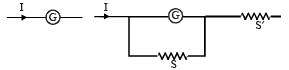QUESTION: 12

A square loop, carrying a steady current I, is placed in a horizontal plane near a long straight conductor carrying a steady current I1 at a distance d from the conductor as shown in figure. The loop will experience    [2011M]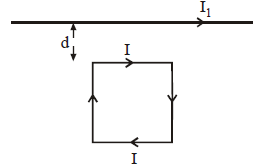Solution: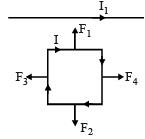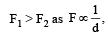and F3 and F4 are equal and opposite. Hence, the net attraction force will be towards the conductor.

QUESTION: 13

Charge q is uniformly spread on a thin ring of radius R. The ring rotates about its axis with a uniform frequency f Hz. The magnitude of magnetic induction at the centre of the ring is        [2011M]

Solution:

When the ring rotates about its axis with a uniform frequency f Hz, the current flowing in the ring is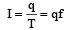Magnetic field at the centre of the ring is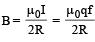QUESTION: 14

Two similar coils of radius R are lying concentrically with their planes at right angles to each other. The currents flowing in them are
I and 2 I, respectively. The resultant magnetic field induction at the centre will be:      

Solution: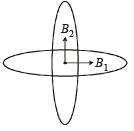The magnetic field, due the coil, carrying current I Ampere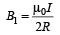The magnetic field due to the coil, carrying current 2I Ampere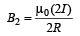The resultant B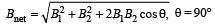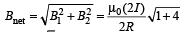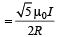QUESTION: 15

An alternating electric field, of frequency v, is applied across the dees (radius = R) of a cyclotron that is being used to accelerate
protons (mass = m). The operating magnetic field (B) used in the cyclotron and the kinetic energy (K) of the proton beam, produced by it, are given by : 

Solution:

Time period of cyclotron is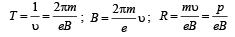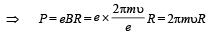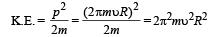QUESTION: 16

An α-particle moves in a circular path of radius   0.83 cm in the presence of a magnetic field of  0.25 Wb/m2. The de-Broglie wavelengthassociated with the particle will be :  

Solution: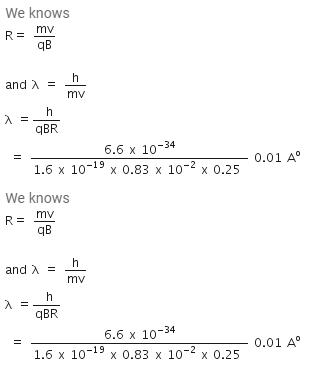QUESTION: 17

A proton carrying 1 MeV kinetic energy ismoving in a circular path of radius R in uniformmagnetic field. What should be the energy of an α -particle to describe a circle of same radius inthe same field?    [2012M]

Solution:

According to the principal of circular motion in a magnetic field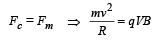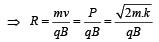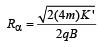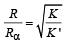but    R = Rα (given)

Thus K = K' = 1 MeV

QUESTION: 18

A current loop in a magnetic field [NEET 2013]

Solution:

A current loop in a magnetic field is in equilibrium in two orientations one is stable and another unstable.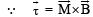= M B sinθ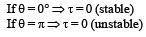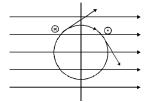Do not experience a torque in some orientations Hence option (c) is correct.

QUESTION: 19

When a proton is released from rest in a room, it starts with an initial acceleration a0 towards west. When it is projected towards north with a speed v0 it moves with an initial acceleration 3a0 towards west. The electric and magnetic fields in the room are respectively      [NEET 2013]

Solution: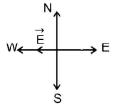When moves with an acceleration a0 towards west, electric field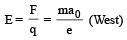When moves with an acceleration 3a0 towards east, magnetic field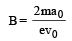(downward)

QUESTION: 20

A long straight wire carries a certain current and produces a magnetic field of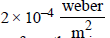at a perpendicular distance of 5 cm from the wire. An electron situated at 5 cm from the wire moves with a velocity 107 m/s towards the wire along perpendicular to it. The force experienced by the electron will be
(charge on electron =1.6 × 10–19 C)

Solution:

Given: Magnetic field B = 2 × 10–4 weber/m2
Velocity of electron, v = 107  m/s
Lorentz force F = qvB sinθ = 1.6 × 10–19 × 107 × 2 × 10–4 (∵ θ = 90°)
= 3.2 × 10–16 N